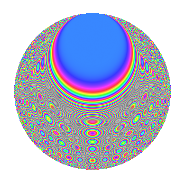# Properties

 Label 2016.2.dfLevel 2016 Weight 2 Character orbit df Rep. character $$\chi_{2016}(929,\cdot)$$ Character field $$\Q(\zeta_{6})$$ Dimension 192 Sturm bound 768

# Related objects

## Defining parameters

 Level: $$N$$ $$=$$ $$2016 = 2^{5} \cdot 3^{2} \cdot 7$$ Weight: $$k$$ $$=$$ $$2$$ Character orbit: $$[\chi]$$ $$=$$ 2016.df (of order $$6$$ and degree $$2$$) Character conductor: $$\operatorname{cond}(\chi)$$ $$=$$ $$63$$ Character field: $$\Q(\zeta_{6})$$ Sturm bound: $$768$$

## Dimensions

The following table gives the dimensions of various subspaces of $$M_{2}(2016, [\chi])$$.

Total New Old
Modular forms 800 192 608
Cusp forms 736 192 544
Eisenstein series 64 0 64

## Trace form

 $$192q + O(q^{10})$$ $$192q + 16q^{21} + 192q^{25} - 24q^{29} + 24q^{45} - 16q^{57} + 48q^{65} + 48q^{77} + 8q^{81} - 72q^{89} + 32q^{93} + O(q^{100})$$

## Decomposition of $$S_{2}^{\mathrm{new}}(2016, [\chi])$$ into newform subspaces

The newforms in this space have not yet been added to the LMFDB.

## Decomposition of $$S_{2}^{\mathrm{old}}(2016, [\chi])$$ into lower level spaces

$$S_{2}^{\mathrm{old}}(2016, [\chi]) \cong$$ $$S_{2}^{\mathrm{new}}(63, [\chi])$$$$^{\oplus 6}$$$$\oplus$$$$S_{2}^{\mathrm{new}}(126, [\chi])$$$$^{\oplus 5}$$$$\oplus$$$$S_{2}^{\mathrm{new}}(252, [\chi])$$$$^{\oplus 4}$$$$\oplus$$$$S_{2}^{\mathrm{new}}(504, [\chi])$$$$^{\oplus 3}$$$$\oplus$$$$S_{2}^{\mathrm{new}}(1008, [\chi])$$$$^{\oplus 2}$$

## Hecke characteristic polynomials

There are no characteristic polynomials of Hecke operators in the database